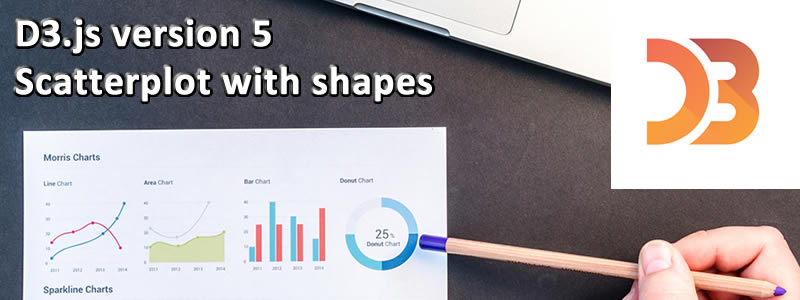# D3.js version 5 Scatterplot with shapesToday I write about how you can create a scatter plot with different shapes in D3.js version 5.

## Aim of this tutorial

This blog builds on Mike Bostocks Scatterplot with shapes example and reworks it for D3.js version 5. This tutorial will focus on the changes needed to convert the original diagram to one that D3.js version 5 supports.

## Preparing some styles for the graph

Before drawing the SVG we need to prepare some styles for the graphs. The following styles are the same as Mike’s original styles for D3 version 3.

```<style>
body {
font: 10px sans-serif;
}

.axis path,
.axis line {
fill: none;
stroke: #000;
shape-rendering: crispEdges;
}

.point {
fill: steelblue;
stroke: #000;
}
</style>```

## Drawing the Scatter Plot

To start with Mike created a number of variables to hold the margin information, width, and height of the graph.

```var margin = {top: 20, right: 20, bottom: 30, left: 40},
width = 960 - margin.left - margin.right,
height = 500 - margin.top - margin.bottom;```

Then the `x` and `y` scales are created and their range is set based on the width and height of the graph. This will determine how the domain values given to the axis are mapped to the size of the graph.

This is one of the changes needed for D3.js version 5, previously scale linear was accessed using `d3.scale.linear()` instead of `d3.scaleLinear()` .

```var x = d3.scaleLinear()
.range([0, width]);
var y = d3.scaleLinear()
.range([height, 0]);```

Then the SVG is added to the body of the page and sized as appropriate. Once we have the SVG the main grouping element `g` is created and transformed into the middle of the SVG.

```var svg = d3.select("body").append("svg")
.attr("width", width + margin.left + margin.right)
.attr("height", height + margin.top + margin.bottom)
.append("g")
.attr("transform", "translate(" + margin.left + "," + margin.top + ")");```

Another change needed for d3 version 5 was the `csv()` function. This now uses a promise structure to return the data using `.then()` after calling csv.

```d3.csv("data.csv")
.then(function(data) {
// Coerce the strings to numbers.
data.forEach(function(d) {
d.x = +d.x;
d.y = +d.y;
});

// Compute the scales’ domains.
x.domain(d3.extent(data, function(d) { return d.x; })).nice();
y.domain(d3.extent(data, function(d) { return d.y; })).nice();

svg.append("g")
.attr("class", "x axis")
.attr("transform", "translate(0," + height + ")")
.call(d3.axisBottom(x));

svg.append("g")
.attr("class", "y axis")
.call(d3.axisLeft(y));

svg.selectAll(".point")
.data(data)
.enter().append("path")
.attr("class", "point")
.attr("d", d3.symbol().type(d3.symbolTriangle))
.attr("transform", function(d) { return "translate(" + x(d.x) + "," + y(d.y) + ")"; });
});```

Inside the csv promise first we convert the x y points to integers, this is because they are strings when they are loaded from the csv function.

Once this is completed we calculate the domains of both of the axis and use `nice()` to ensure they start and end at nice values. Once the domains are calculated we create both the y and x axis and add it to the SVG.

Again we have to modify this slightly from D3.js version 3 so `d3.svg.axis().scale(x).orient("bottom")` becomes `d3.axisBottom(x)`, and `d3.svg.axis().scale(y).orient("left")` becomes `d3.axisLeft(y)`.

The final section of code creates each of the points and sets the `d` attribute to the triangle symbol. This has changed from `d3.svg.symbol().type("triangle-up")` in D3.js version 3 to `d3.symbol().type(d3.symbolTriangle)`.

## Summary

Today I went through the code to create a scatter plot with D3.js version 5 by updating Mike Bostocks version 3 graph. This includes showing how to have custom shapes with your scatter plot and the version 5 changes.

The full code for this is available on my website which includes a live example.

If you have any questions feel free to comment on the following blog post.